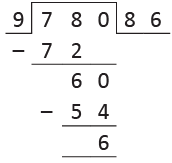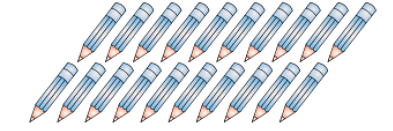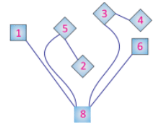Olympiad Test Level 2: Computing Operations

# Olympiad Test Level 2: Computing Operations

Test Description

## 15 Questions MCQ Test Mathematics Olympiad for Class 3 | Olympiad Test Level 2: Computing Operations

Olympiad Test Level 2: Computing Operations for Class 3 2023 is part of Mathematics Olympiad for Class 3 preparation. The Olympiad Test Level 2: Computing Operations questions and answers have been prepared according to the Class 3 exam syllabus.The Olympiad Test Level 2: Computing Operations MCQs are made for Class 3 2023 Exam. Find important definitions, questions, notes, meanings, examples, exercises, MCQs and online tests for Olympiad Test Level 2: Computing Operations below.
 1 Crore+ students have signed up on EduRev. Have you?
Olympiad Test Level 2: Computing Operations - Question 1

### The product of two numbers is 2,979. If one of the numbers is 9, the difference between the two numbers is ____________.

Detailed Solution for Olympiad Test Level 2: Computing Operations - Question 1

Product = 2,979

One number = 9

Other number = 2,979 ÷ 9 = 331

Difference between the two

numbers = 331 – 9 = 322

Olympiad Test Level 2: Computing Operations - Question 2

### Maximum 4 boys can sit on a bench. How many benches are required for 432 boys to sit ?

Detailed Solution for Olympiad Test Level 2: Computing Operations - Question 2

Total boys = 432
Number of boys on each bench = 4
Total number of benches needed = 432 ÷ 4 = 108 benches

Olympiad Test Level 2: Computing Operations - Question 3

### The remainder when 780 is divided by 9 is ____________.

Detailed Solution for Olympiad Test Level 2: Computing Operations - Question 3Olympiad Test Level 2: Computing Operations - Question 4

Arrange the following pencils in groups in such a way that each group can contain 5 pencils. How many such groups will be formed ?Detailed Solution for Olympiad Test Level 2: Computing Operations - Question 4

Total pencils = 20

Number of pencils in each group = 5

Number of groups = 20 ÷ 5 = 4

Olympiad Test Level 2: Computing Operations - Question 5

Ashima participated in a charity fair for a week. She had a stall of toys in the fair. Ashima sold 123 toy cars, 89 dolls and 100 balls in a week. How many toys did Ashima sell in all ?

Detailed Solution for Olympiad Test Level 2: Computing Operations - Question 5

Total number of toys sold = 123 + 89 + 100 = 312

Olympiad Test Level 2: Computing Operations - Question 6

The difference between the smallest and the greatest four digit number formed by the given digits, using each digit only once is _________.

9 7 8 5

Detailed Solution for Olympiad Test Level 2: Computing Operations - Question 6

Greatest number of

four digits that can be formed is 9,875.

Smallest number of four digits that can be formed is 5,789.

Difference = 9,875 – 5,789 = 4,086

Olympiad Test Level 2: Computing Operations - Question 7

The cost of 5 mobile phones is ₹45,000. What is the cost of 12 such mobile phones ?

Detailed Solution for Olympiad Test Level 2: Computing Operations - Question 7

Cost of 5 mobile phones = ₹ 45,000

Cost of 1 mobile phone

= ₹ 45,000 ÷ 5 = ₹ 9,000

Cost of 12 mobile phones

= ₹ 9,000 × 12 = ₹ 108,000

Olympiad Test Level 2: Computing Operations - Question 8

Riya’s room has 5 shelves to keep books. Each shelf can have 13 books. If Riya has 82 books, then how many books will be left after arranging the books in 5 shelves ?

Detailed Solution for Olympiad Test Level 2: Computing Operations - Question 8

Total books = 82

Books that can be arranged on shelves = 5 × 13 = 65

Number of books left = 82 – 65 = 17

Olympiad Test Level 2: Computing Operations - Question 9

Kavya bought 12 dozen bananas. She gave 5 dozen bananas to her brother and used 8 bananas to make a shake. How many bananas were left with her ?

Detailed Solution for Olympiad Test Level 2: Computing Operations - Question 9

Total bananas = 12 dozens

Bananas given to brother = 5 dozens

Bananas left = 12 dozens – 5 dozens

= 7 dozens = 7×12 = 84

Bananas used for making a shake = 8

Bananas left in the end = 84 – 8 = 76

Olympiad Test Level 2: Computing Operations - Question 10

During a discount sale on a shop, 197 customers visit on the first day, 220 more customers visit on the second day than the first day. How many customers visit the shop on both days together ?

Detailed Solution for Olympiad Test Level 2: Computing Operations - Question 10

Number of customers on first day = 197

Number of customers on second day = 197 + 220 = 417

Total number of visitors on both days = 197 + 417 = 614

Olympiad Test Level 2: Computing Operations - Question 11

Rohan distributed ₹ 9,876 equally among 12 children. How much did each child get ?

Detailed Solution for Olympiad Test Level 2: Computing Operations - Question 11

Each child gets

₹ 9,876 ÷ 12 = ₹ 823

Olympiad Test Level 2: Computing Operations - Question 12

The sum of a mystery number and 8,900 is 100 less than 9,500. What is the mystery number ?

Detailed Solution for Olympiad Test Level 2: Computing Operations - Question 12

Mystery number +8,900 = 9,500 – 100 = 9,400

⇒ Mystery number = 9,400 – 8,900

= 500

Olympiad Test Level 2: Computing Operations - Question 13

Riya, Seema and Sonia bought some books. Sonia bought 10 books. Riya bought 5 more books than Sonia. Seema bought 3 more books than Riya. How many books did they buy in all ?

Detailed Solution for Olympiad Test Level 2: Computing Operations - Question 13

Number of books bought by Sonia = 10 books

Number of books bought by Riya

= 10 + 5 = 15 books

Number of books bought by Seema

= 15 + 3 = 18 books

Total number of books bought by them = 10 + 15 + 18 = 43 books

Olympiad Test Level 2: Computing Operations - Question 14

In the following figure, Alex multiplied the greatest and the smallest number and then he added the rest of the numbers to the product. Find the result.Detailed Solution for Olympiad Test Level 2: Computing Operations - Question 14

Largest number = 8

Smallest number = 1

Product = 8 × 1 = 8

Sum = 8 + 5 + 2 + 3 + 4 + 6 = 28

Olympiad Test Level 2: Computing Operations - Question 15

A reporter wrote a report, which was published in a newspaper. It has 26 words in each line. If the report has 58 lines, how many words are there in all the reports ?

Detailed Solution for Olympiad Test Level 2: Computing Operations - Question 15

Number of words in each line = 26
Number of lines = 58
Total number of words = 26 × 58
= 1,508

## Mathematics Olympiad for Class 3

26 videos|26 docs|32 tests(Scan QR code)